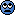# Prerequisites for non Euclidean geometry

Hi, i would be very interested to start learning hyperbolic geometry, what would be the necessary prerequisites to begin it's study?A good familiarity with vector calculus and basic linear algebra should suffice.

The reason I didn't mention hyperbolic geometry specifically is that vector calculus and linear algebra prepare one for a basis course on the differential geometry of curves and surfaces in 3-space. This is just what one would need to understand hyperbolic geometry in the right context (even though a complete hyperbolic plane of constant Gaussian curvature = -1 cannot be embedded in 3-space if it is of differentiability class C2. — Hilbert's theorem).

Hyperbolic geometry is ideally learned as an advanced topic in a course on the differential geometry of curves and surfaces in 3-space. So, the prerequisites are for such a course.

Hence so it would be necessary to learn before some notion of differential geometry of surfaces in 3d-space!

Hence so it would be necessary to learn before some notion of differential geometry of surfaces in 3d-space!

No, it is not necessary. Differential hyperbolic geometry is a subset of hyperbolic geometry, but definitely not the only part.

Thank you for helping me solve this doubt!It is possible that micromass and I have different viewpoints about this issue. I won't say that differential geometry is absolutely necessary if all you want to learn is hyperbolic geometry.

But in my opinion it would greatly enhance your understanding, and would be an extremely wise thing to learn either before or during the time you learn about hyperbolic geometry.